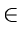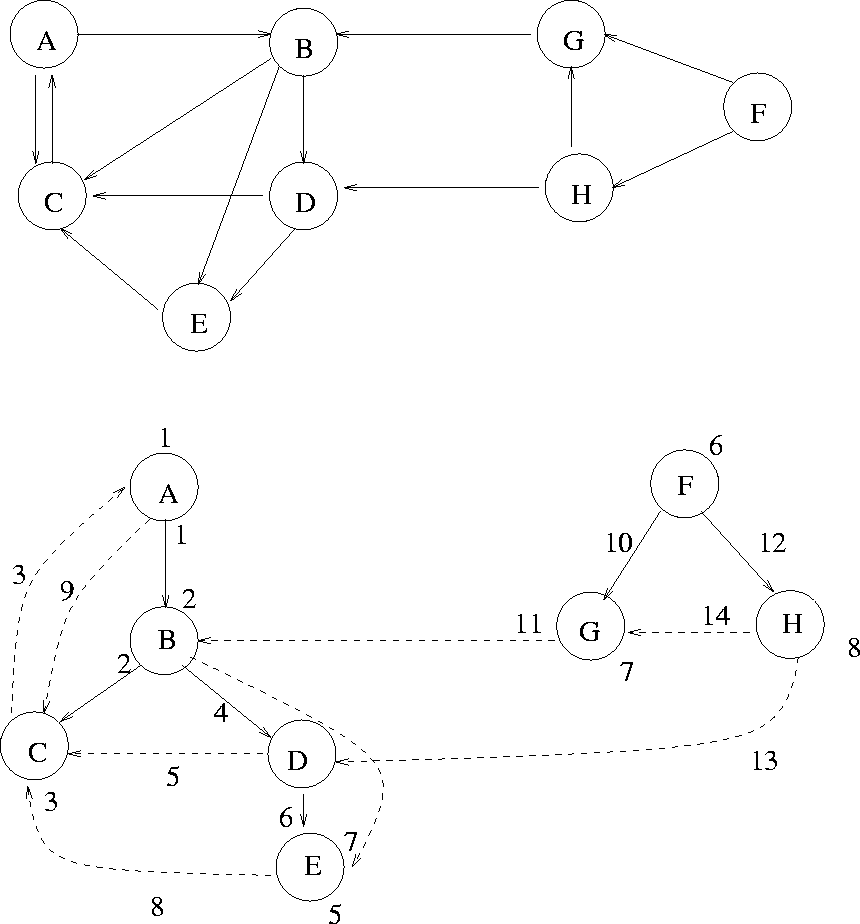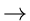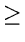Next: 7.4.1 Breadth First Search Up: 7. Directed Graphs Previous: 7.3 Warshall's Algorithm

# 7.4 Depth First Search and Breadth First Search

• An important way of traversing all vertices of a digraph, with applications in many problems.
• Let L[v] be the adjacency list for vertex v. To do a depth first search of all vertices emanating from v, we have the following recursive scheme:

void dfs (vertex v)

{

vertex w;

mark v as visited;

for each vertex wL[v]

dfs(w)

}• To do a dfs on the entire digraph, we first unmark all vertices and do a dfs on all unmarked vertices:

{

for vV

mark v as unvisited;

for vV

if (v is unvisited)

dfs(v);

}

Example: See Figure 7.10.

DFS Arcs:

• Tree Arcs: ab, N(a) < N(b) leading to unvisited vertices
• Non-Tree Arcs:
• back arcs: ab, N(a)N(b), b is an ancestor
• forward arcs: ab, N(a) < N(b), b is a proper descendant
• cross arcs: ab. Neither ancestor nor descendantNext: 7.4.1 Breadth First Search Up: 7. Directed Graphs Previous: 7.3 Warshall's Algorithm
eEL,CSA_Dept,IISc,Bangalore